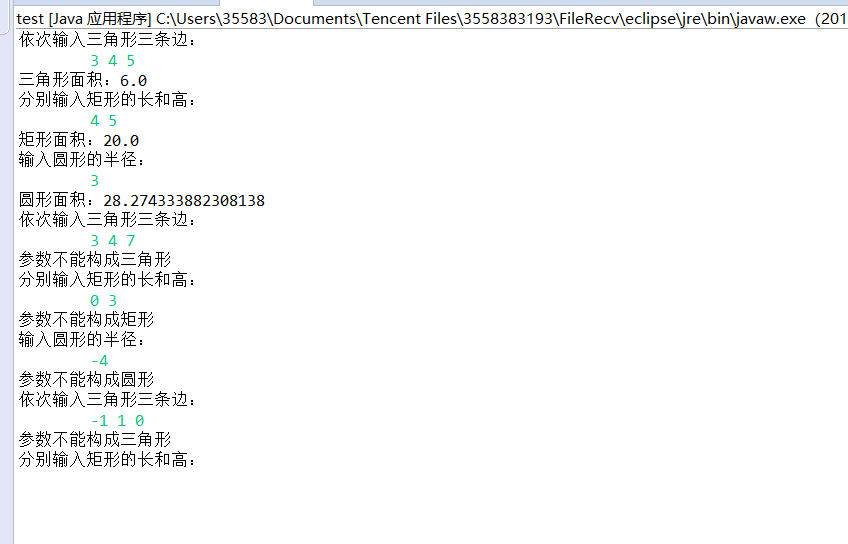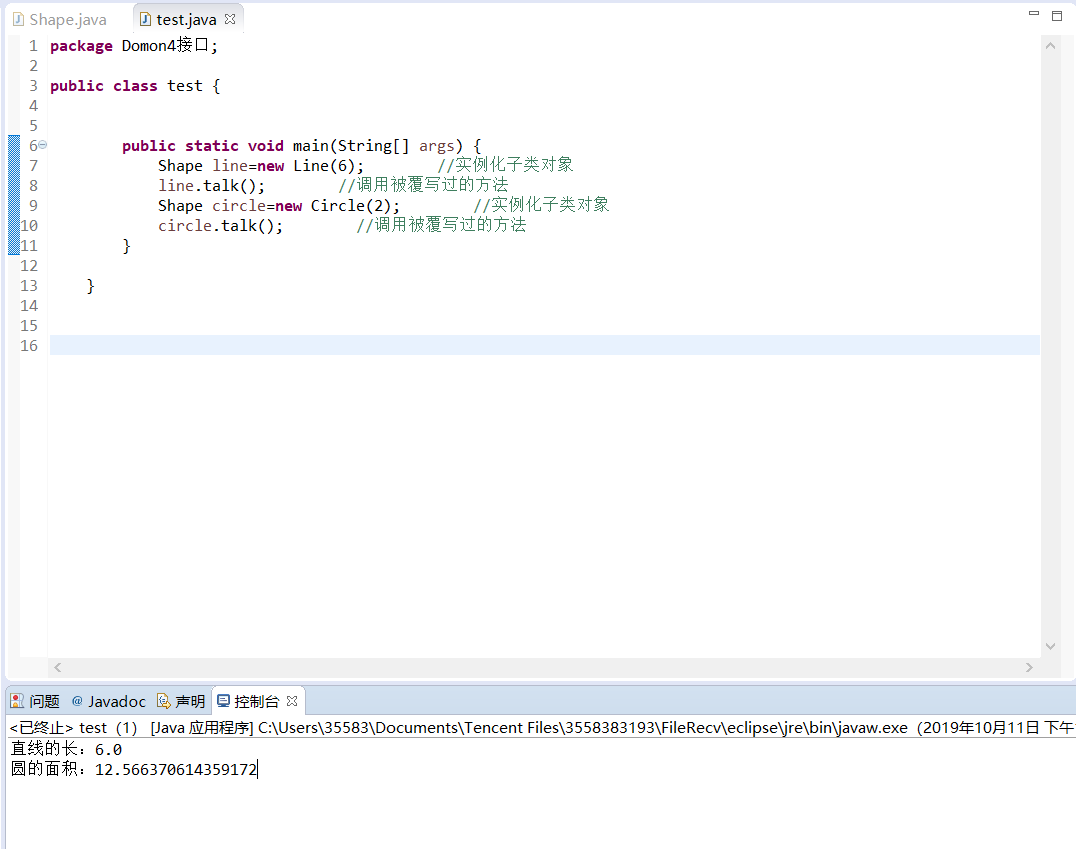# Java实验报告

## 实验四 类的继承

### 实验内容

（一）抽象类的使用

1. 设计一个类层次，定义一个抽象类--形状，其中包括有求形状的面积的抽象方法。 继承该抽象类定义三角型、矩形、圆。 分别创建一个三角形、矩形、圆存对象，将各类图形的面积输出。
注：三角形面积s=sqrt(p(p-a)(p-b)*(p-c)) 其中，a,b,c为三条边，p=(a+b+c)/2
2.编程技巧

(1) 抽象类定义的方法在具体类要实现；

(2) 使用抽象类的引用变量可引用子类的对象；

(3) 通过父类引用子类对象，通过该引用访问对象方法时实际用的是子类的方法。可将所有对象存入到父类定义的数组中。
（二）使用接口技术
1定义接口Shape，其中包括一个方法size（）,设计"直线"、"圆"、类实现Shape接口。分别创建一个"直线"、"圆"对象，将各类图形的大小输出。

(1) 接口中定义的方法在实现接口的具体类中要重写实现；

(2) 利用接口类型的变量可引用实现该接口的类创建的对象。

（一）抽象类的使用

### （1）实验代码

``````package Domon4;

public abstract class Shape {
public abstract void talkArea();
}

class Triangle extends Shape{
private double a,b,c,p; //三角形三条边和公式常量

public Triangle(int a,int b,int c){
if(a>0&&b>0&&c>0&&a+b>c&&a+c>b&&b+c>a){ //判断参数是否满足构成三角形的条件
this.a=a;
this.b=b;
this.c=c;
}
else{
System.out.println("参数不能构成三角形");
}
}

public double getA() {
return a;
}

public double getB() {
return b;
}

public double getC() {
return c;
}

public void talkArea() {
p=(a+b+c)/2;
if(a>0&&b>0&&c>0&&a+b>c&&a+c>b&&b+c>a) //判断参数是否满足构成三角形的条件
System.out.println("三角形面积："+ Math.sqrt(p*(p-a)*(p-b)*(p-c)));
}
}

class Rectangle extends Shape{
private double length,hight; //矩形的长和宽

public Rectangle(double length,double hight){
if(length>0&&hight>0){ //判断参数是否满足构成矩形的条件
this.hight=hight;
this.length=length;
}
else{
System.out.println("参数不能构成矩形");
}
}
public double getLength() {
return length;
}

public double getHight() {
return hight;
}

public void talkArea() {
if(length>0&&hight>0) //判断参数是否满足构成三角形的条件
System.out.println("矩形面积："+length*hight);
}

}

class Circle extends Shape{

}
else{
System.out.println("参数不能构成圆形");
}
}

}

public void talkArea() {
}

}``````
``````package Domon4;

import java.util.Scanner;

public class test {

public static void main(String[] args) {

for(int i=0;i<100;i++){
Scanner input=new Scanner(System.in);
System.out.println("依次输入三角形三条边：");
int a=input.nextInt();
int b=input.nextInt();
int c=input.nextInt();
Shape Triangle = new Triangle(a, b, c); //通过父类引用子类对象
Triangle.talkArea();

System.out.println("分别输入矩形的长和高：");
int length=input.nextInt();
int hight=input.nextInt();
Shape Rectangle = new Rectangle(length,hight); //通过父类引用子类对象
Rectangle.talkArea();

System.out.println("输入圆形的半径：");
int r=input.nextInt();
Shape Circle = new Circle(r); //通过父类引用子类对象
Circle.talkArea();
}
}
}``````

### （2）运行结果（二）使用接口技术

### （1）实验代码

``````package Domon4接口;

public interface Shape {
public abstract double size();
public abstract  void talk();
}

class Line implements Shape {
public double length;        //直线的长度

Line(double length){ //定义构造方法
this.length=length;
}

public double size() {        //覆写接口Shape中的抽象方法
return length;
}

public void talk() { //覆写接口Shape中的抽象方法
System.out.println("直线的长："+size());

}

}

class Circle implements Shape {

}

public double size() {        //覆写接口Shape中的抽象方法
}

public void talk() {        //覆写接口Shape中的抽象方法
System.out.println("圆的面积："+size());

}

}``````
``````package Domon4接口;

public class test {

public static void main(String[] args) {
Shape line=new Line(6);        //实例化子类对象
line.talk();        //调用被覆写过的方法
Shape circle=new Circle(2);        //实例化子类对象
circle.talk();        //调用被覆写过的方法
}

} ``````

### （2）运行结果### 课程总结

（1）本次实验属于抽象类和接口的学习，感觉内容还挺不错，对于应用性可能会比较实用，学起来感觉掌握有限，需要继续深层次学习。

（2）本周学习了抽象类的基本概念、接口的基本概念、对象的多态性、instanceof关键字、抽象类abstract和接口的应用中的为抽象类与接口实例化、抽象类的实际应用——模板实际、接口的实际应用——制定标准，都在课堂上有了初步的认识，还需课余巩固深入，当然后面还介绍了几种设计模式——工厂设计、代理设计、适配器设计等等。

（1）向上转型：子类对象---->父类对象（自动转型）。
（2）向下转型：父类对象---->子类对象（强制转型）

（1）object关键词：Object类是一个特殊的类，是所有类的父类，如果一个类没有用extends明确指出继承于某个类，那么它默认继承Object类。
（2）object类的主要方法
(2.1)toString();
(2.2)equals();
(2.3) hashCode()；